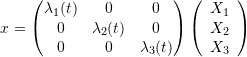### Displacement and Strain: The Velocity Gradient

The Velocity Gradient is a spacial tensor that carries the information on the velocity of vectors in the deformed configuration when an object is being deformed as a function of time. Let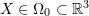describe the position in the reference configuration and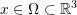describe the instantaneous position in the deformed configuration. The velocity field of the deformed configuration is described by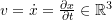. Let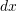be a vector in the deformed configuration, being the image of a vector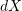in the reference configuration. Then, the rate of change ofwith respect to time, namely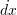is given by: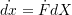The relationship between the vectors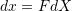, can be used to replaceas follows: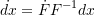That way, the vectoris a function of the vector. The tensor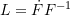is termed the velocity gradient since it is the gradient of the vector fieldas follows.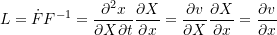In component form, the velocity gradient has the form: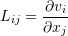The stretching tensor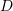is defined as the symmetric part of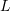while the spin tensor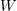is defined as the skewsymmetric part of: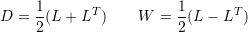### Behaviour under Rigid Body Rotation:

The stretching tensor is a true measure of the instantaneous deformation of an object. Assuming that a body is moving in space with a rigid body rotation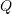, i.e.: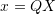Then, the velocity gradient is equal to: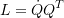The stretching and spin tensors have the following forms: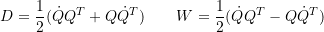However, the rotation matrix satisfies the following relationships: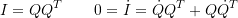Therefore: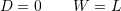### Local Change in Volume and the Velocity Gradient

An important relationship that is used throughout the derivations in continuum mechanics is the relationship between the trace of the velocity gradient, namely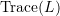and the determinant of the deformation gradient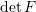. Denoting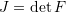, the relationship is given as follows: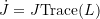There are two ways to show the above relationship. The first relies on expressing each side in terms of their components and is adopted from the book by Ogden.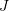can be expressed in terms of the components of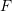as follows: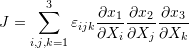Then, by taking the time derivatives: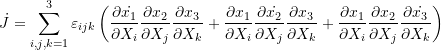The following relationship is first used to replace the expressions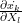with the velocity gradient components: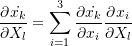From the properties of the determinant, the following expressions are equal to zero for any values of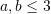: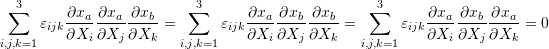Which results in the following: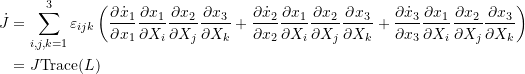Another proof is adopted from the book by P. Chadwick and relies on the expressions shown in the matrix invariants section. Let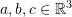be three linearly independent vectors.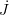can be expressed as follows: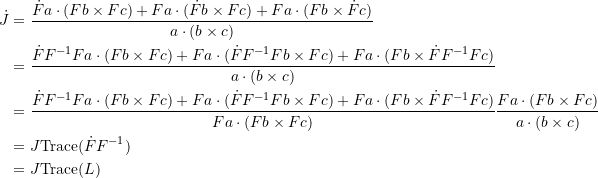The above relationship implies that if a deformation is associated with a zero change in volume, i.e.,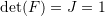and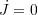, then this is associated with a zero trace of the velocity gradient. i.e., for an isochoric motion: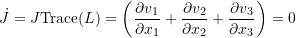### Examples and Problems:

#### Problems:

1. Consider the two dimensional position function: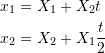Evaluate the following at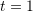:,,,, and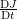. Also, findat which the above relationship will stop being physically possible.

2. Find the stretch and spin tensors of the deformation described by: